# Problem 3: Heart Disease Classification

This is the accompanying document to the heart disease classification report for the computational intelligence coursework by student 10011412. It details the process taken in modelling and gaining insights into the heart disease data.

## Formatting Data

Initially, we will first import the data into R and label it

### Training Data

trainD <- read.table("C:/Users/Manny/Documents/Manny/Studies/Uni 4th yr - 2nd Tri/CI/CourseWork/NN/cwTRAIN.txt",
header = FALSE)
colnames(trainD) <- c("Age", "Sex", "CPType", "RestingBP", "SerumChol", "FBS",
"RestingECG", "MaxHR", "EIA", "STDep", "PES", "BloodVesNo", "thal", "HD")
summary(trainD)

##       Age            Sex            CPType       RestingBP     SerumChol
##  Min.   :29.0   Min.   :0.000   Min.   :1.00   Min.   : 94   Min.   :126
##  1st Qu.:47.8   1st Qu.:0.000   1st Qu.:3.00   1st Qu.:120   1st Qu.:213
##  Median :55.0   Median :1.000   Median :3.00   Median :130   Median :243
##  Mean   :54.4   Mean   :0.668   Mean   :3.16   Mean   :131   Mean   :249
##  3rd Qu.:61.0   3rd Qu.:1.000   3rd Qu.:4.00   3rd Qu.:140   3rd Qu.:275
##  Max.   :77.0   Max.   :1.000   Max.   :4.00   Max.   :200   Max.   :564
##       FBS         RestingECG       MaxHR          EIA
##  Min.   :0.00   Min.   :0.00   Min.   : 71   Min.   :0.000
##  1st Qu.:0.00   1st Qu.:0.00   1st Qu.:132   1st Qu.:0.000
##  Median :0.00   Median :2.00   Median :154   Median :0.000
##  Mean   :0.15   Mean   :1.03   Mean   :149   Mean   :0.345
##  3rd Qu.:0.00   3rd Qu.:2.00   3rd Qu.:166   3rd Qu.:1.000
##  Max.   :1.00   Max.   :2.00   Max.   :202   Max.   :1.000
##      STDep           PES         BloodVesNo         thal
##  Min.   :0.00   Min.   :1.00   Min.   :0.000   Min.   :3.00
##  1st Qu.:0.00   1st Qu.:1.00   1st Qu.:0.000   1st Qu.:3.00
##  Median :0.65   Median :2.00   Median :0.000   Median :3.00
##  Mean   :1.01   Mean   :1.59   Mean   :0.682   Mean   :4.66
##  3rd Qu.:1.65   3rd Qu.:2.00   3rd Qu.:1.000   3rd Qu.:7.00
##  Max.   :5.60   Max.   :3.00   Max.   :3.000   Max.   :7.00
##        HD
##  Min.   :0.000
##  1st Qu.:0.000
##  Median :1.000
##  Mean   :0.564
##  3rd Qu.:1.000
##  Max.   :1.000


### Testing Data


testD <- read.table("C:/Users/Manny/Documents/Manny/Studies/Uni 4th yr - 2nd Tri/CI/CourseWork/NN/cwTEST.txt",
header = FALSE)
colnames(testD) <- c("Age", "Sex", "CPType", "RestingBP", "SerumChol", "FBS",
"RestingECG", "MaxHR", "EIA", "STDep", "PES", "BloodVesNo", "thal", "HD")
summary(testD)

##       Age            Sex           CPType       RestingBP     SerumChol
##  Min.   :35.0   Min.   :0.00   Min.   :1.00   Min.   :110   Min.   :164
##  1st Qu.:48.2   1st Qu.:0.00   1st Qu.:3.00   1st Qu.:120   1st Qu.:214
##  Median :55.5   Median :1.00   Median :3.00   Median :134   Median :256
##  Mean   :54.5   Mean   :0.72   Mean   :3.22   Mean   :135   Mean   :254
##  3rd Qu.:61.0   3rd Qu.:1.00   3rd Qu.:4.00   3rd Qu.:144   3rd Qu.:286
##  Max.   :71.0   Max.   :1.00   Max.   :4.00   Max.   :180   Max.   :353
##       FBS         RestingECG     MaxHR          EIA           STDep
##  Min.   :0.00   Min.   :0    Min.   : 97   Min.   :0.00   Min.   :0.00
##  1st Qu.:0.00   1st Qu.:0    1st Qu.:138   1st Qu.:0.00   1st Qu.:0.25
##  Median :0.00   Median :1    Median :153   Median :0.00   Median :1.10
##  Mean   :0.14   Mean   :1    Mean   :151   Mean   :0.26   Mean   :1.23
##  3rd Qu.:0.00   3rd Qu.:2    3rd Qu.:162   3rd Qu.:0.75   3rd Qu.:1.60
##  Max.   :1.00   Max.   :2    Max.   :190   Max.   :1.00   Max.   :6.20
##       PES         BloodVesNo        thal            HD
##  Min.   :1.00   Min.   :0.00   Min.   :3.00   Min.   :0.00
##  1st Qu.:1.00   1st Qu.:0.00   1st Qu.:3.00   1st Qu.:0.00
##  Median :1.50   Median :0.00   Median :3.00   Median :1.00
##  Mean   :1.56   Mean   :0.62   Mean   :4.84   Mean   :0.52
##  3rd Qu.:2.00   3rd Qu.:1.00   3rd Qu.:7.00   3rd Qu.:1.00
##  Max.   :3.00   Max.   :3.00   Max.   :7.00   Max.   :1.00


### Converting to categorical variables

Some values within the data are categorical, we shall label them as such and ensure that they are unordered. This will prevent bias in the model and the tree will more accurately fit the data.

We will then print out the structure of our data frames(objects).

Not that PES is stored as an ordinal variable since it is an ordered categorical variable.


trainD$Sex <- as.factor(trainD$Sex)
trainD$CPType <- as.factor(trainD$CPType)
trainD$FBS <- as.factor(trainD$FBS)
trainD$RestingECG <- as.factor(trainD$RestingECG)
trainD$EIA <- as.factor(trainD$EIA)
trainD$PES <- as.ordered(trainD$PES)
trainD$thal <- as.factor(trainD$thal)
trainD$HD <- as.factor(trainD$HD)

str(trainD)

## 'data.frame':    220 obs. of  14 variables:
##  $Age : num 70 67 57 64 74 65 56 59 60 63 ... ##$ Sex       : Factor w/ 2 levels "0","1": 2 1 2 2 1 2 2 2 2 1 ...
##  $CPType : Factor w/ 4 levels "1","2","3","4": 4 3 2 4 2 4 3 4 4 4 ... ##$ RestingBP : num  130 115 124 128 120 120 130 110 140 150 ...
##  $SerumChol : num 322 564 261 263 269 177 256 239 293 407 ... ##$ FBS       : Factor w/ 2 levels "0","1": 1 1 1 1 1 1 2 1 1 1 ...
##  $RestingECG: Factor w/ 3 levels "0","1","2": 3 3 1 1 3 1 3 3 3 3 ... ##$ MaxHR     : num  109 160 141 105 121 140 142 142 170 154 ...
##  $EIA : Factor w/ 2 levels "0","1": 1 1 1 2 2 1 2 2 1 1 ... ##$ STDep     : num  2.4 1.6 0.3 0.2 0.2 0.4 0.6 1.2 1.2 4 ...
##  $PES : Ord.factor w/ 3 levels "1"<"2"<"3": 2 2 1 2 1 1 2 2 2 2 ... ##$ BloodVesNo: num  3 0 0 1 1 0 1 1 2 3 ...
##  $thal : Factor w/ 3 levels "3","6","7": 1 3 3 3 1 3 2 3 3 3 ... ##$ HD        : Factor w/ 2 levels "0","1": 1 2 1 2 2 2 1 1 1 1 ...


Lets do the same for the the test data

testD$Sex <- as.factor(testD$Sex)
testD$CPType <- as.factor(testD$CPType)
testD$FBS <- as.factor(testD$FBS)
testD$RestingECG <- as.factor(testD$RestingECG)
testD$EIA <- as.factor(testD$EIA)
testD$PES <- as.ordered(testD$PES)
testD$thal <- as.factor(testD$thal)
testD$HD <- as.factor(testD$HD)

str(testD)

## 'data.frame':    50 obs. of  14 variables:
##  $Age : num 54 65 57 63 35 41 62 43 58 52 ... ##$ Sex       : Factor w/ 2 levels "0","1": 2 2 2 2 1 2 1 1 1 2 ...
##  $CPType : Factor w/ 4 levels "1","2","3","4": 4 4 3 4 4 2 3 4 1 1 ... ##$ RestingBP : num  110 135 150 130 138 135 130 132 150 118 ...
##  $SerumChol : num 239 254 168 330 183 203 263 341 283 186 ... ##$ FBS       : Factor w/ 2 levels "0","1": 1 1 1 2 1 1 1 2 2 1 ...
##  $RestingECG: Factor w/ 2 levels "0","2": 1 2 1 2 1 1 1 2 2 2 ... ##$ MaxHR     : num  126 127 174 132 182 132 97 136 162 190 ...
##  $EIA : Factor w/ 2 levels "0","1": 2 1 1 2 1 1 1 2 1 1 ... ##$ STDep     : num  2.8 2.8 1.6 1.8 1.4 0 1.2 3 1 0 ...
##  $PES : Ord.factor w/ 3 levels "1"<"2"<"3": 2 2 1 1 1 2 2 2 1 2 ... ##$ BloodVesNo: num  1 1 0 3 0 0 1 0 0 0 ...
##  $thal : Factor w/ 3 levels "3","6","7": 3 3 1 3 1 2 3 3 1 2 ... ##$ HD        : Factor w/ 2 levels "0","1": 1 1 2 1 2 2 1 1 2 2 ...


So looks like our data is finally prepared. Lets print out a summary and view our data.

### Data Distribution


summary(trainD)

##       Age       Sex     CPType    RestingBP     SerumChol   FBS
##  Min.   :29.0   0: 73   1: 18   Min.   : 94   Min.   :126   0:187
##  1st Qu.:47.8   1:147   2: 33   1st Qu.:120   1st Qu.:213   1: 33
##  Median :55.0           3: 64   Median :130   Median :243
##  Mean   :54.4           4:105   Mean   :131   Mean   :249
##  3rd Qu.:61.0                   3rd Qu.:140   3rd Qu.:275
##  Max.   :77.0                   Max.   :200   Max.   :564
##  RestingECG     MaxHR     EIA         STDep      PES       BloodVesNo
##  0:106      Min.   : 71   0:144   Min.   :0.00   1:105   Min.   :0.000
##  1:  2      1st Qu.:132   1: 76   1st Qu.:0.00   2:100   1st Qu.:0.000
##  2:112      Median :154           Median :0.65   3: 15   Median :0.000
##             Mean   :149           Mean   :1.01           Mean   :0.682
##             3rd Qu.:166           3rd Qu.:1.65           3rd Qu.:1.000
##             Max.   :202           Max.   :5.60           Max.   :3.000
##  thal    HD
##  3:126   0: 96
##  6: 10   1:124
##  7: 84
##
##
##

View(trainD)

summary(testD)

##       Age       Sex    CPType   RestingBP     SerumChol   FBS
##  Min.   :35.0   0:14   1: 2   Min.   :110   Min.   :164   0:43
##  1st Qu.:48.2   1:36   2: 9   1st Qu.:120   1st Qu.:214   1: 7
##  Median :55.5          3:15   Median :134   Median :256
##  Mean   :54.5          4:24   Mean   :135   Mean   :254
##  3rd Qu.:61.0                 3rd Qu.:144   3rd Qu.:286
##  Max.   :71.0                 Max.   :180   Max.   :353
##  RestingECG     MaxHR     EIA        STDep      PES      BloodVesNo
##  0:25       Min.   : 97   0:37   Min.   :0.00   1:25   Min.   :0.00
##  2:25       1st Qu.:138   1:13   1st Qu.:0.25   2:22   1st Qu.:0.00
##             Median :153          Median :1.10   3: 3   Median :0.00
##             Mean   :151          Mean   :1.23          Mean   :0.62
##             3rd Qu.:162          3rd Qu.:1.60          3rd Qu.:1.00
##             Max.   :190          Max.   :6.20          Max.   :3.00
##  thal   HD
##  3:26   0:24
##  6: 4   1:26
##  7:20
##
##
##

View(testD)


It looks like the 'Sex' and 'FBS' training data is heavily biased. Additionally, the Resting ECG '1' category is not represented in the test set.

So lets get started by looking at the distribution of some of our key data points in the training set:

hist(trainD$Age)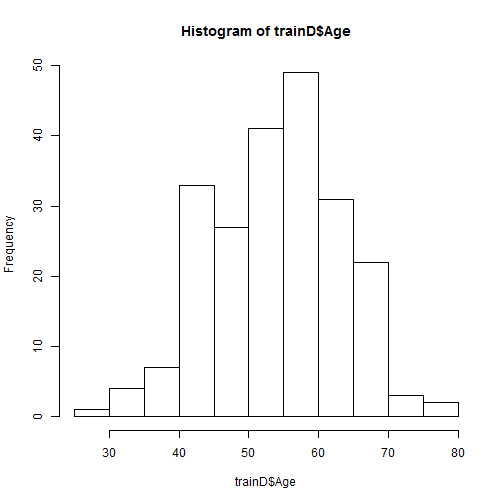It looks like the majority of the patients in the test set are between 40 and 70 years old. So now have a feeling of how our data behaves. Keeping this in mind, Lets attempt to classify heart disease using some tree-based methods. ## Decision Trees We initially attempt to classify using the simplest tree-based model, the decision tree. So lets attach our dataset to the workspace so that we can easily call its attributes. You may need to install the tree package #install.packages(tree). We then fit a tree to this data with the response variable set to the “Heart Disease” category. Finally, we plot the decision tree and view its detailed summary. set.seed(2) require(tree)  ## Loading required package: tree  attach(trainD) tree.trainD = tree(HD ~ ., data = trainD) plot(tree.trainD) text(tree.trainD, pretty = 0)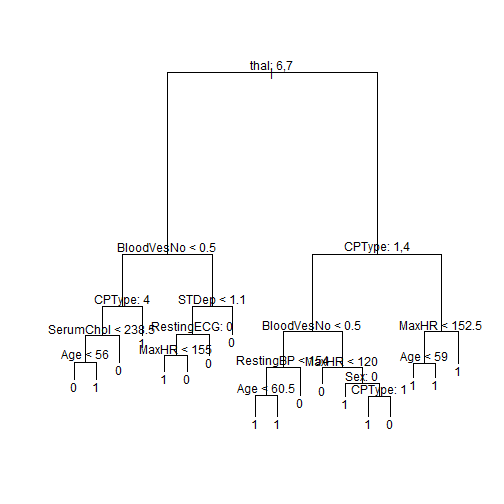tree.trainD  ## node), split, n, deviance, yval, (yprob) ## * denotes terminal node ## ## 1) root 220 300 1 ( 0.44 0.56 ) ## 2) thal: 6,7 94 100 0 ( 0.73 0.27 ) ## 4) BloodVesNo < 0.5 42 60 0 ( 0.52 0.48 ) ## 8) CPType: 4 25 30 0 ( 0.72 0.28 ) ## 16) SerumChol < 238.5 15 20 0 ( 0.53 0.47 ) ## 32) Age < 56 9 10 0 ( 0.78 0.22 ) * ## 33) Age > 56 6 5 1 ( 0.17 0.83 ) * ## 17) SerumChol > 238.5 10 0 0 ( 1.00 0.00 ) * ## 9) CPType: 1,2,3 17 20 1 ( 0.24 0.76 ) * ## 5) BloodVesNo > 0.5 52 30 0 ( 0.90 0.10 ) ## 10) STDep < 1.1 22 20 0 ( 0.77 0.23 ) ## 20) RestingECG: 0 12 20 0 ( 0.58 0.42 ) ## 40) MaxHR < 155 5 5 1 ( 0.20 0.80 ) * ## 41) MaxHR > 155 7 6 0 ( 0.86 0.14 ) * ## 21) RestingECG: 2 10 0 0 ( 1.00 0.00 ) * ## 11) STDep > 1.1 30 0 0 ( 1.00 0.00 ) * ## 3) thal: 3 126 100 1 ( 0.21 0.79 ) ## 6) CPType: 1,4 54 70 1 ( 0.43 0.57 ) ## 12) BloodVesNo < 0.5 31 30 1 ( 0.23 0.77 ) ## 24) RestingBP < 154 26 20 1 ( 0.12 0.88 ) ## 48) Age < 60.5 17 0 1 ( 0.00 1.00 ) * ## 49) Age > 60.5 9 10 1 ( 0.33 0.67 ) * ## 25) RestingBP > 154 5 5 0 ( 0.80 0.20 ) * ## 13) BloodVesNo > 0.5 23 30 0 ( 0.70 0.30 ) ## 26) MaxHR < 120 6 0 0 ( 1.00 0.00 ) * ## 27) MaxHR > 120 17 20 0 ( 0.59 0.41 ) ## 54) Sex: 0 5 5 1 ( 0.20 0.80 ) * ## 55) Sex: 1 12 10 0 ( 0.75 0.25 ) ## 110) CPType: 1 5 7 1 ( 0.40 0.60 ) * ## 111) CPType: 4 7 0 0 ( 1.00 0.00 ) * ## 7) CPType: 2,3 72 30 1 ( 0.06 0.94 ) ## 14) MaxHR < 152.5 20 20 1 ( 0.20 0.80 ) ## 28) Age < 59 12 20 1 ( 0.33 0.67 ) * ## 29) Age > 59 8 0 1 ( 0.00 1.00 ) * ## 15) MaxHR > 152.5 52 0 1 ( 0.00 1.00 ) *  The length of the legs of a tree branch is proportional to the significance of the splitting variable. As can be seen, thal, CPType and BloodVesNo are the most significant variables. Lets see how good the tree is at predicting the values it was trained on. We will represent the results in a simple misclassification table and then calculate the classification rate. tree.pred = predict(tree.trainD, trainD, type = "class") with(trainD, table(tree.pred, HD))  ## HD ## tree.pred 0 1 ## 0 80 4 ## 1 16 120  (80 + 120)/220  ##  0.9091  We have a base classification rate of 91% on the data that the tree was trained on. So lets now predict on the test data using this decision tree and see what we get. We will represent the results in a simple misclassification table and then calculate the classification rate. tree.pred = predict(tree.trainD, testD[, c(1:13)], type = "class") with(testD, table(tree.pred, testD$HD))

##
## tree.pred  0  1
##         0 18  1
##         1  6 25

(18 + 25)/50

##  0.86


As can be seen, our classification rate drops down to 86% when using the basic decision tree against the unseen test data.

The problem is that this tree is probably too complex and is overfitting the data .

We have to remember that the summary and histograms (above) of the data made it very clear that the training data is biased so we need to simplify the model in order to provide for better classification on unseen data sets.

Simpler models are always better.

#### Cross-Validation for tree pruning

We have one tuning parameter with the decision tree which is the depth of the tree.

To simplify the model, we are going to 'prune' the branches of the tree. We will use cross-validation to decide the depth at which the branches will be pruned. So basically, cross validation will be used to determine the size and complexity of the decision tree.

The method cv.tree, does 10-fold cross-validation by default. We will then prune the tree according to the misclassification rate.

Cross Validation should always be used to choose values for tuning parameters

cv.trainD = cv.tree(tree.trainD, FUN = prune.misclass)
cv.trainD

## $size ##  18 15 11 9 7 6 2 1 ## ##$dev
##  53 54 54 55 56 58 61 96
##
## $k ##  -Inf 0.0 1.0 1.5 2.0 3.0 4.5 44.0 ## ##$method
##  "misclass"
##
## attr(,"class")
##  "prune"         "tree.sequence"

plot(cv.trainD)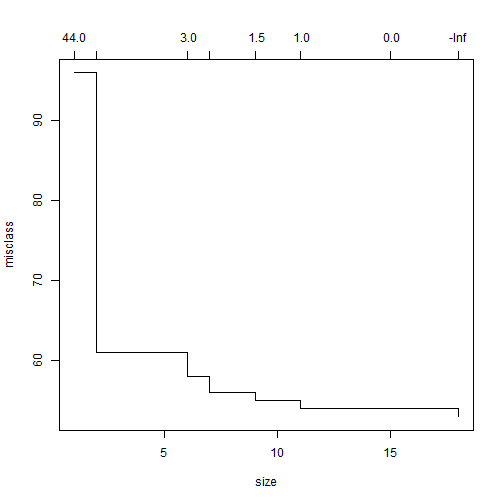From the plot of the misclassification rate against the size of the tree (the number of nodes/leafes at the bottom of the tree). We see that we can prune the tree down significantly and reduce its complexity by a factor of two (from 18 leaf nodes to 9) without performing significantly worse on the training data.

General rule of thumb in statistics is to always choose a simpler model, as long as it is within one standard deviation of the best model. This reduces the model bias.

So lets test the new tree on our training data.

prune.trainD = prune.misclass(tree.trainD, best = 9)
tree.pred = predict(prune.trainD, trainD, type = "class")
with(trainD, table(tree.pred, HD))

##          HD
## tree.pred   0   1
##         0  84  15
##         1  12 109

(84 + 109)/220

##  0.8773


Our classification rate against the training data used to build the tree has dropped from 91% to 88%.

Lets see how that compares against the unseen test data, using the new classification tree.

tree.pred = predict(prune.trainD, testD[, c(1:13)], type = "class")
with(testD, table(tree.pred, testD$HD))  ## ## tree.pred 0 1 ## 0 19 1 ## 1 5 25  (19 + 25)/50  ##  0.88  The simpler tree performs better than the original tree on the test data. Our classification rate has increased from 86% to 88%. Let's plot out new tree plot(prune.trainD) text(prune.trainD, pretty = 0)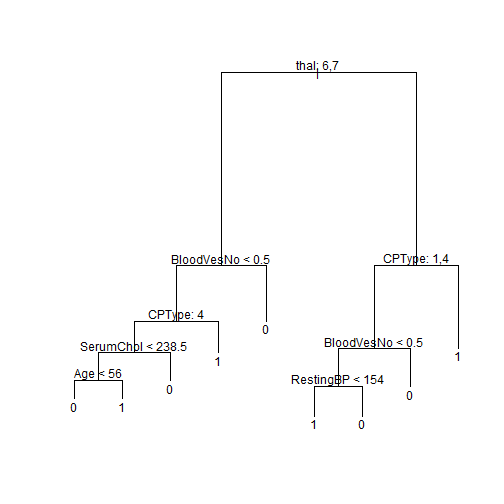As can be seen, this tree is alot simpler. It only uses 6 of the 13 variables and performs better than the tree that used all the variables. So pruning with cross-validation gave us a simpler yet more accurate tree. ## Random Forests Let us now fit a basic random forest to our data. You may need to install the tree package #install.packages(randomForest). set.seed(2) require(randomForest)  ## Loading required package: randomForest ## randomForest 4.6-7 ## Type rfNews() to see new features/changes/bug fixes.   rf.trainD = randomForest(HD ~ ., data = trainD)  Lets use this forest to predict on the training data. rf.pred = predict(rf.trainD, trainD) with(trainD, table(rf.pred, trainD$HD))

##
## rf.pred   0   1
##       0  96   0
##       1   0 124

(96 + 124)/220

##  1


As can be seen, we obtain 100% classification on the data used to train the random forest. This behaviour is expected of random forests applied to their training data. One of the advantages of random forests is that they don't over fit the data.

Lets predict using our random forest on the unseen test set.

Note that we are adding the missing category from restingECG in the first line below. This is needed for the randomForest package to work. It cannot predict on data with differrent levels to the variables.

levels(testD$RestingECG) <- "1" rf.pred = predict(rf.trainD, testD[, c(1:13)]) with(testD, table(rf.pred, testD$HD))

##
## rf.pred  0  1
##       0 20  1
##       1  4 25

(20 + 25)/50

##  0.9


We can see that the basic random forest performs better than our best decision tree on the test data set. It has a classification rate of 90% on the data.

The problem is that the random forest is probably way to complex for this type of data set. Lets first reduce the number of trees being grown in this forest.

plot(rf.trainD, log = "y")
legend("top", colnames(rf.trainD$err.rate), col = 1:4, cex = 0.8, fill = 1:4)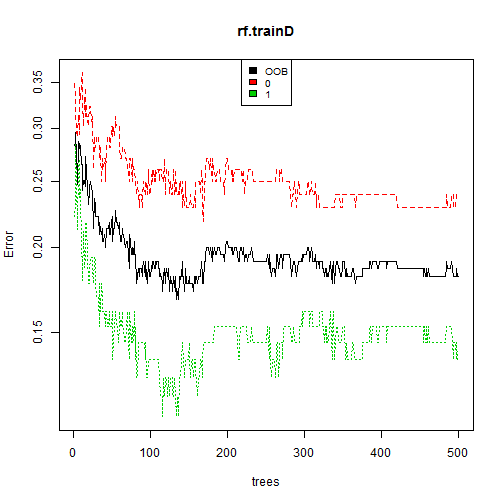As can be seen, the average Out-Of-Bag (OOB) error doesn't seem to improve much after we the tree count goes above 120. So we shall limit the tree count to 110. rf.trainD = randomForest(HD ~ ., data = trainD, importance = TRUE, ntree = 110) rf.trainD = randomForest(HD ~ ., data = trainD, ntree = 110, do.trace = 10)  ## ntree OOB 1 2 ## 10: 21.56% 28.72% 16.13% ## 20: 21.36% 27.08% 16.94% ## 30: 21.82% 23.96% 20.16% ## 40: 20.45% 22.92% 18.55% ## 50: 18.64% 21.88% 16.13% ## 60: 20.00% 23.96% 16.94% ## 70: 20.00% 25.00% 16.13% ## 80: 20.00% 25.00% 16.13% ## 90: 19.55% 26.04% 14.52% ## 100: 19.09% 25.00% 14.52% ## 110: 17.73% 25.00% 12.10%  rf.pred = predict(rf.trainD, testD[, c(1:13)]) with(testD, table(rf.pred, testD$HD))

##
## rf.pred  0  1
##       0 20  1
##       1  4 25

(20 + 25)/50

##  0.9


With our random forest, we can now get back up to 90% classification rate using a simpler tree.

The original random forest was more complex, it used 500 trees.

The final random forest uses only 110 trees but it performs as well as the 500-tree forest and classifies 90% of unseen test data.

We gained an improvement of 2% using a random forests over decision trees.

Random Forests are a black box technique and there is no way of predicting how they will behave.

So, lets see what made this forest good at classifying the data.

plot(rf.trainD, log = "y")
legend("top", colnames(rf.trainD$err.rate), col = 1:4, cex = 0.8, fill = 1:4)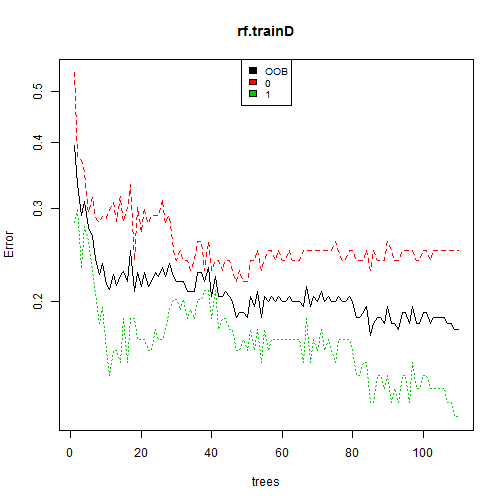rf.trainD$importance

##            MeanDecreaseGini
## Age                  8.8435
## Sex                  3.0150
## CPType              13.3204
## RestingBP            9.6944
## SerumChol            8.8971
## FBS                  0.8224
## RestingECG           2.0544
## MaxHR               13.6824
## EIA                  4.8880
## STDep                9.8055
## PES                  4.9971
## BloodVesNo          13.7218
## thal                12.9828


As can be seen, the importance of any variable to a particular sex doesnt seem to vary much when compared to the other sex.

When we look at the 'MeanDecreaseAccuracy', which measures how important a variable is at reducing the classification error rate, we see that the 'thal', 'BloodVesNo' and 'CPType' are the most important variables. This is similar to what we observed in the decision tree.

Additionally, if we look at the 'MeanDecreaseGini', which measure how important each variable is at separating the data, we see again that 'thal', 'BloodVesNo' and 'CPType' are very important. But so are 'STDep' and 'MaxHR'.

## Cross Validation for Random Forest

Random forests also have one last parameter that we can tune.

It is called “mtry”, it is basically the number of predictors sampled by each splitting node. It's default value is 3.

We will use cross-validation to pick this parameter.

You may need to install the caret package #install.packages(“caret”). If caret doesn't behave as expected, you may be missing one of its 21 dependacies.

You can install them through #install.packages(“caret”, dependencies = c(“Depends”, “Suggests”))

THIS IS A LONG INSTALL ~15 minutes

library(caret)

## Loading required package: lattice
## Loading required package: ggplot2

set.seed(20)

controller = trainControl(method = "cv", number = 10, repeats = 1, returnResamp = "final")

cv.rf.trainD = train(HD ~ ., data = trainD, method = "rf", trControl = controller,
ntree = 110, importance = TRUE, tuneLength = 17)

cv.rf.pred = predict(cv.rf.trainD, testD[, c(1:13)])

with(testD, table(cv.rf.pred, testD$HD))  ## ## cv.rf.pred 0 1 ## 0 20 1 ## 1 4 25  (20 + 25)/50  ##  0.9  10-fold Cross-validation on the mtry parameter doesn't seem to have improved the classification rate. That means that the default value was a good value to use. Lets see details for this model cv.rf.trainD$finalModel

##
## Call:
##  randomForest(x = x, y = y, ntree = 110, mtry = param$mtry, importance = TRUE) ## Type of random forest: classification ## Number of trees: 110 ## No. of variables tried at each split: 3 ## ## OOB estimate of error rate: 20% ## Confusion matrix: ## 0 1 class.error ## 0 71 25 0.2604 ## 1 19 105 0.1532  This basically re-iterates the default values the 'mtry' parameter. We have the same OOB and the same mtry. The only difference is that this was achieved via cross-validation. Lets look in detail at how the values of mtry varied and what significance they had. cv.rf.trainD  ## Random Forest ## ## 220 samples ## 13 predictors ## 2 classes: '0', '1' ## ## No pre-processing ## Resampling: Cross-Validated (10 fold) ## ## Summary of sample sizes: 199, 199, 198, 198, 197, 198, ... ## ## Resampling results across tuning parameters: ## ## mtry Accuracy Kappa Accuracy SD Kappa SD ## 2 0.8 0.6 0.1 0.3 ## 3 0.8 0.6 0.1 0.3 ## 4 0.8 0.6 0.1 0.2 ## 5 0.8 0.6 0.1 0.3 ## 6 0.8 0.6 0.1 0.3 ## 7 0.8 0.6 0.1 0.3 ## 8 0.8 0.6 0.1 0.3 ## 9 0.8 0.6 0.2 0.3 ## 10 0.8 0.6 0.1 0.3 ## 10 0.8 0.6 0.1 0.3 ## 10 0.8 0.6 0.1 0.2 ## 10 0.8 0.6 0.1 0.3 ## 10 0.8 0.6 0.1 0.3 ## 20 0.8 0.6 0.1 0.3 ## 20 0.8 0.6 0.1 0.3 ## 20 0.8 0.6 0.1 0.2 ## 20 0.8 0.6 0.1 0.3 ## ## Accuracy was used to select the optimal model using the largest value. ## The final value used for the model was mtry = 3.  All values of mtry have the same accuracy and kappa. This means that the 'mtry' tuning parameter is insignificant for this forest. Now lets see what variables it thought were significant. varImp(cv.rf.trainD)  ## rf variable importance ## ## Importance ## BloodVesNo 100.0 ## thal7 94.4 ## CPType4 87.0 ## STDep 52.2 ## MaxHR 51.4 ## PES.Q 47.8 ## Sex1 44.0 ## PES.L 41.4 ## EIA1 35.7 ## CPType3 34.6 ## thal6 33.5 ## RestingECG2 17.2 ## CPType2 16.9 ## SerumChol 15.1 ## RestingECG1 13.5 ## Age 12.4 ## RestingBP 12.2 ## FBS1 0.0  We see some of the variables that are most significant at separating the data, but in more detail than before. Our data set and variable count is too small to warrant investigation of other classification techiniques such as Boosting ## Cross-Validated - Neural Networks Neural networks are very differrent from the tree-based approaches so far. This neural network has one hidden layer. It has only two tuning parameters (neurons in hidden layer and weight decay). Lets use 10-fold cross validation to select the number of neurons in the hidden layer and weight decay. You may need to install the neural network package nnet. #install.packages(“nnet”) require("nnet")  ## Loading required package: nnet   controller = trainControl(method = "cv", number = 10, repeats = 1, returnResamp = "final") nn.net = train(trainD$HD ~ ., method = "nnet", data = trainD, trControl = controller,
finalModel = T, trace = FALSE)
nn.pred = predict(nn.net, testD[, c(1:13)])
with(testD, table(nn.pred, testD$HD))  ## ## nn.pred 0 1 ## 0 20 3 ## 1 4 23  (20 + 23)/50  ##  0.86  So our best performance on the neural network is 86% percent using a neural network. Lets look at this model in more detail nn.net$bestTune

##   size decay
## 6    3   0.1

nn.net$finalModel  ## a 18-3-1 network with 61 weights ## inputs: Age Sex1 CPType2 CPType3 CPType4 RestingBP SerumChol FBS1 RestingECG1 RestingECG2 MaxHR EIA1 STDep PES.L PES.Q BloodVesNo thal6 thal7 ## output(s): .outcome ## options were - entropy fitting decay=0.1  Our best settings are 3 hidden units and a 0.1 weight decay. Again, if you really need to know the details on how this choice is made then you can look at: plot(nn.net)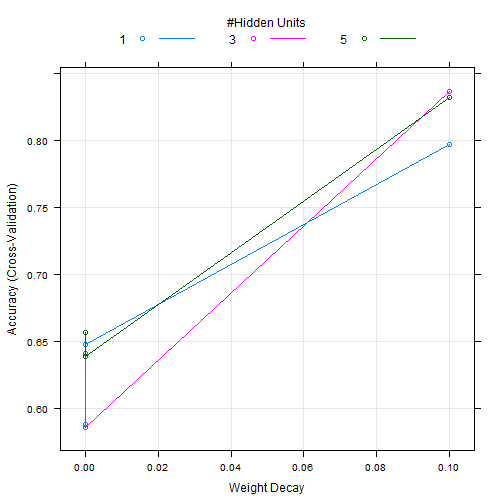nn.net$results

##   size decay Accuracy   Kappa AccuracySD KappaSD
## 1    1 0e+00   0.5876 0.07154    0.07859  0.1892
## 2    1 1e-04   0.6477 0.19979    0.13233  0.3225
## 3    1 1e-01   0.7970 0.57519    0.12207  0.2725
## 4    3 0e+00   0.6405 0.21512    0.11149  0.2760
## 5    3 1e-04   0.5855 0.05418    0.06999  0.1713
## 6    3 1e-01   0.8363 0.66245    0.09912  0.2073
## 7    5 0e+00   0.6561 0.25220    0.13016  0.3112
## 8    5 1e-04   0.6387 0.20945    0.11054  0.2818
## 9    5 1e-01   0.8319 0.65634    0.05326  0.1056


Let us see what our final neural network looks like:

You will need the nnet.plot function : #https://gist.github.com/fawda123/7471137

plot(nn.net$finalModel)  ## Loading required package: scales ## Loading required package: reshape ## Loading required package: plyr ## ## Attaching package: 'reshape' ## ## The following objects are masked from 'package:plyr': ## ## rename, round_any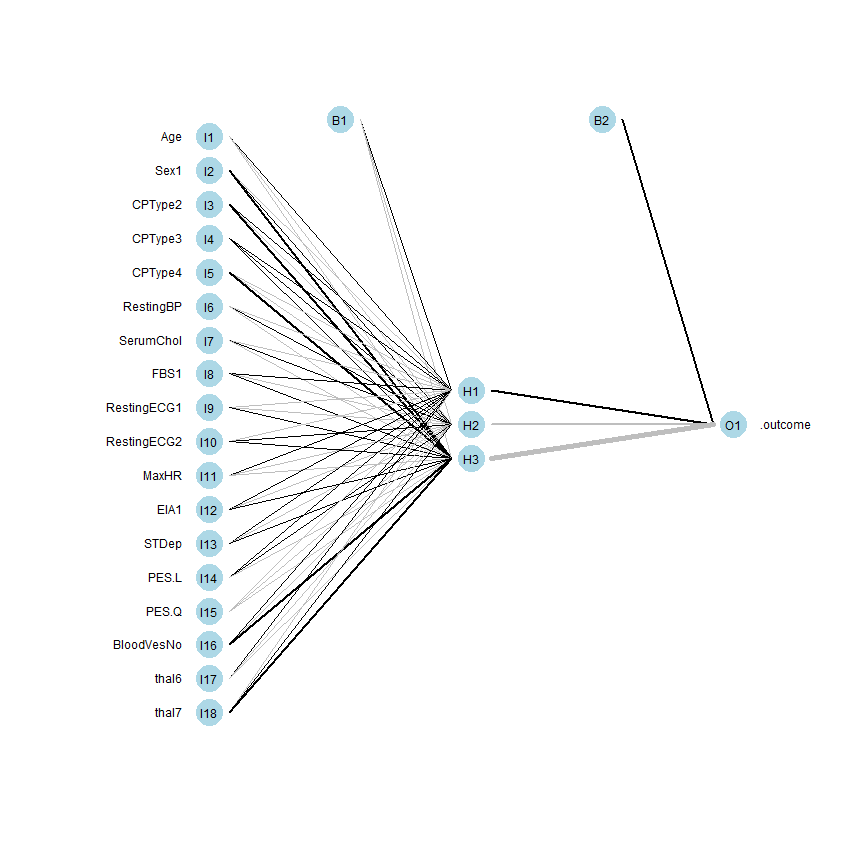There is something peculiar about our neural network. Sex has suddenly become a very important predictor (highlighted by a dark thick line). This is inconsistent with our previous models. ### Normalising the data We need to scale the data, this was not an issue when dealing with decision trees and random forests. But it becomes an issue when dealing with neural networks https://stackoverflow.com/questions/8961586/do-i-need-to-normalize-or-scale-data-for-randomforest-r-package We will basically normalise all real values and retrain the network. trainD$Age <- scale(trainD$Age) trainD$RestingBP <- scale(trainD$RestingBP) trainD$SerumChol <- scale(trainD$SerumChol) trainD$MaxHR <- scale(trainD$MaxHR) trainD$STDep <- scale(trainD$STDep) trainD$BloodVesNo <- scale(trainD$BloodVesNo) testD$Age <- scale(testD$Age) testD$RestingBP <- scale(testD$RestingBP) testD$SerumChol <- scale(testD$SerumChol) testD$MaxHR <- scale(testD$MaxHR) testD$STDep <- scale(testD$STDep) testD$BloodVesNo <- scale(testD$BloodVesNo) controller = trainControl(method = "cv", number = 10, repeats = 1, returnResamp = "final") nn.net = train(trainD$HD ~ ., method = "nnet", data = trainD, trControl = controller,
finalModel = T, trace = FALSE)
nn.pred = predict(nn.net, testD[, c(1:13)])
with(testD, table(nn.pred, testD$HD))  ## ## nn.pred 0 1 ## 0 19 1 ## 1 5 25  (19 + 25)/50  ##  0.88  After rescaling, our classification rate is now up to 88%. nn.net$bestTune

##   size decay
## 3    1   0.1

nn.net$finalModel  ## a 18-1-1 network with 21 weights ## inputs: Age Sex1 CPType2 CPType3 CPType4 RestingBP SerumChol FBS1 RestingECG1 RestingECG2 MaxHR EIA1 STDep PES.L PES.Q BloodVesNo thal6 thal7 ## output(s): .outcome ## options were - entropy fitting decay=0.1  Our model is also much simpler, with only one neuron in the hidden layer and 21 weights. Lets also plot how the differrent values for weight decay affect the accuracy for differrent values of the hidden weights. plot(nn.net)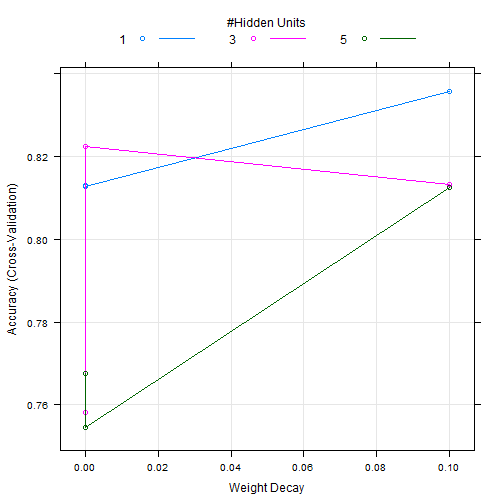nn.net$results

##   size decay Accuracy  Kappa AccuracySD KappaSD
## 1    1 0e+00   0.8129 0.6249    0.08079  0.1577
## 2    1 1e-04   0.8127 0.6281    0.07734  0.1497
## 3    1 1e-01   0.8356 0.6653    0.06069  0.1266
## 4    3 0e+00   0.7581 0.5098    0.07456  0.1490
## 5    3 1e-04   0.8224 0.6398    0.05565  0.1153
## 6    3 1e-01   0.8131 0.6192    0.08597  0.1793
## 7    5 0e+00   0.7676 0.5274    0.06438  0.1348
## 8    5 1e-04   0.7546 0.5028    0.07113  0.1449
## 9    5 1e-01   0.8124 0.6178    0.07658  0.1624


Let us also plot our final neural network structure.

plot(nn.net$finalModel)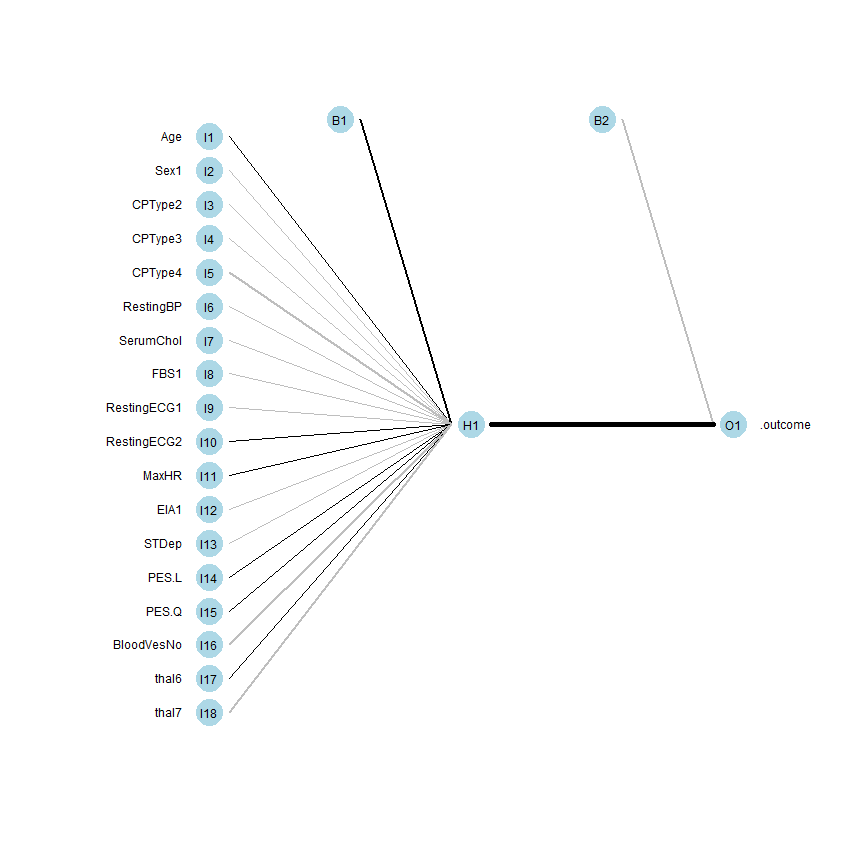Note that the black lines are positive weights while the gray ones are negative. Additionally, there thickness represents there magnitude. We can see that Sex is no longer an overly emphasised predictor. We can also view our weights. plot(nn.net$finalModel, wts.only = T)

## $hidden 1 1 ##  2.82994 0.39039 -1.13632 -1.21786 -0.04779 -2.01690 -0.55280 ##  -0.23061 -0.08214 -0.01823 0.04503 0.72545 -0.44754 -0.33525 ##  0.36162 1.19904 -1.51615 0.03882 -1.71760 ## ##$out 1
##  -2.669  6.000


Note that the first (top) value is always the bias weight. And we just read the weights going into each layer from top to bottom.

With this, we complete our investigation and recommend all of these models for the classifcation of heart disease problem. But we ramain biased towards the tree based methods as they will scale alot better.

End of Investigation.

Copyright © 2014 Emmanuel Kahembwe

#### Add commentRefresh